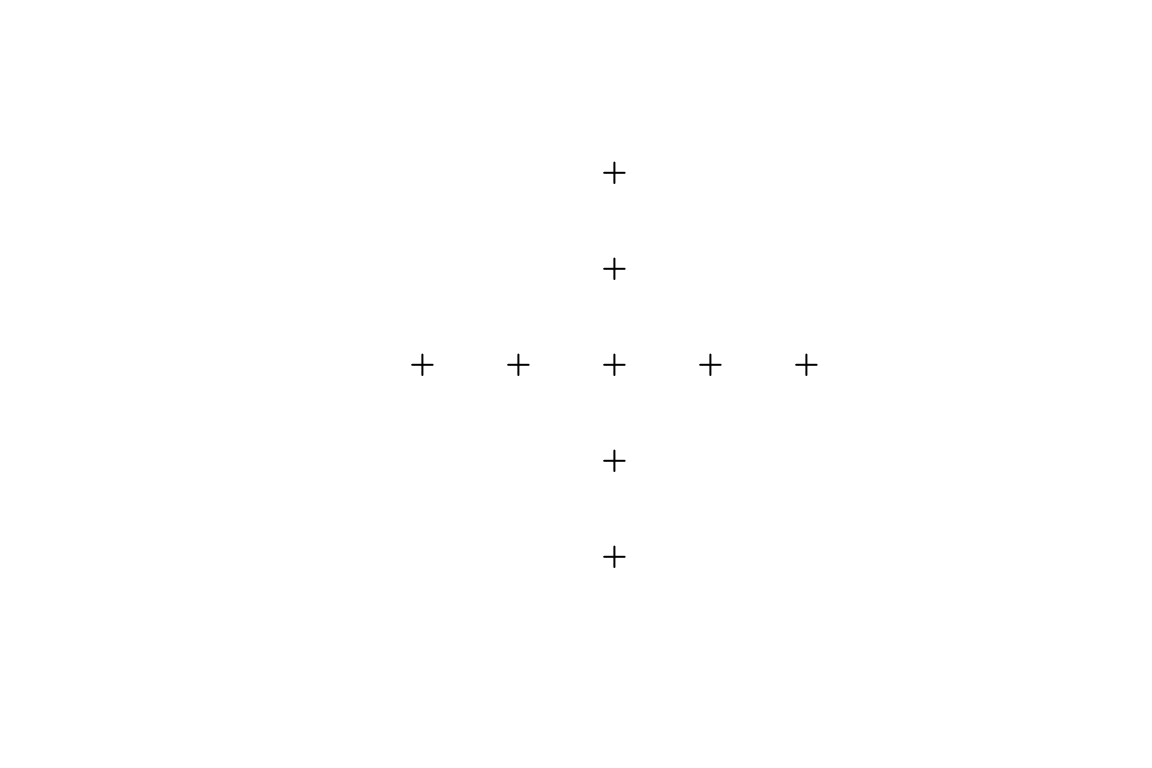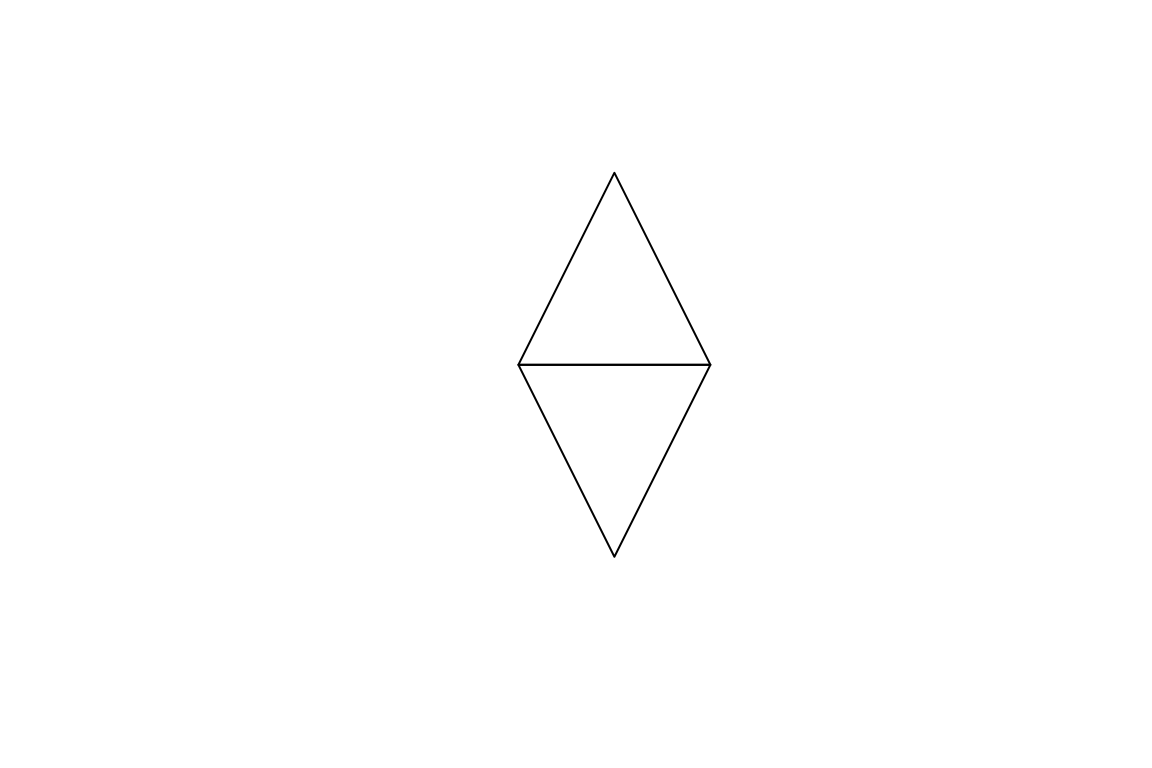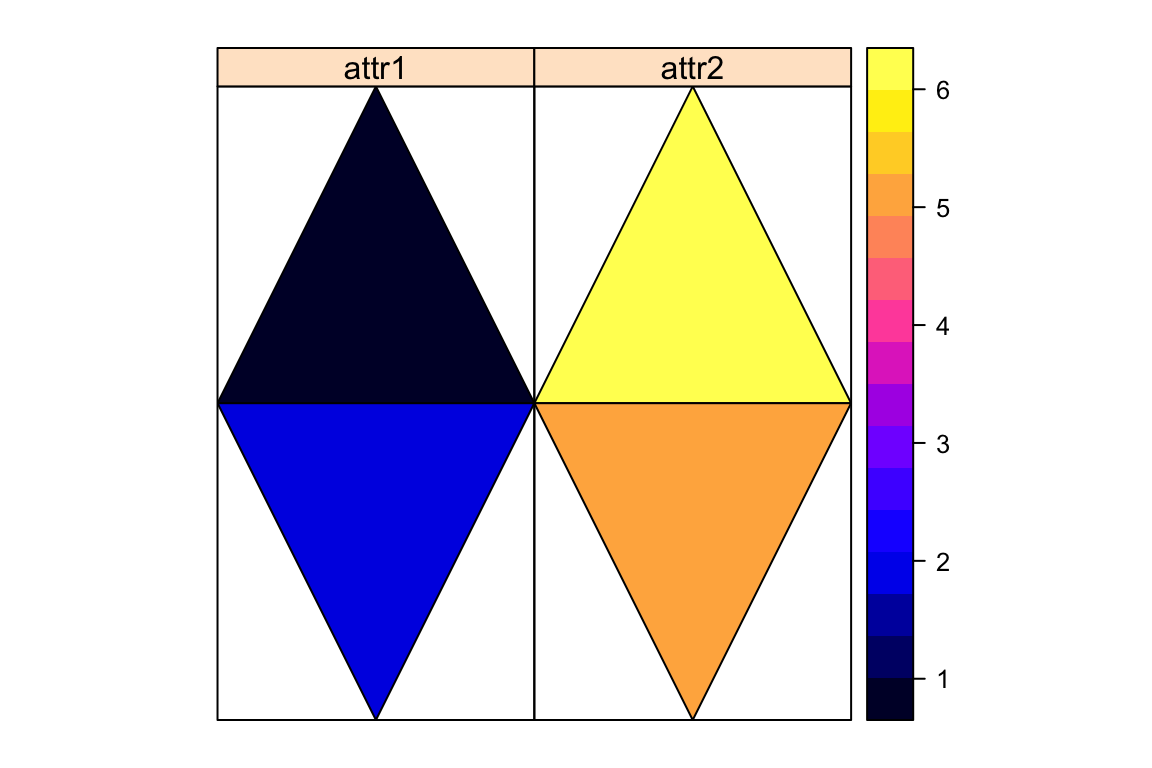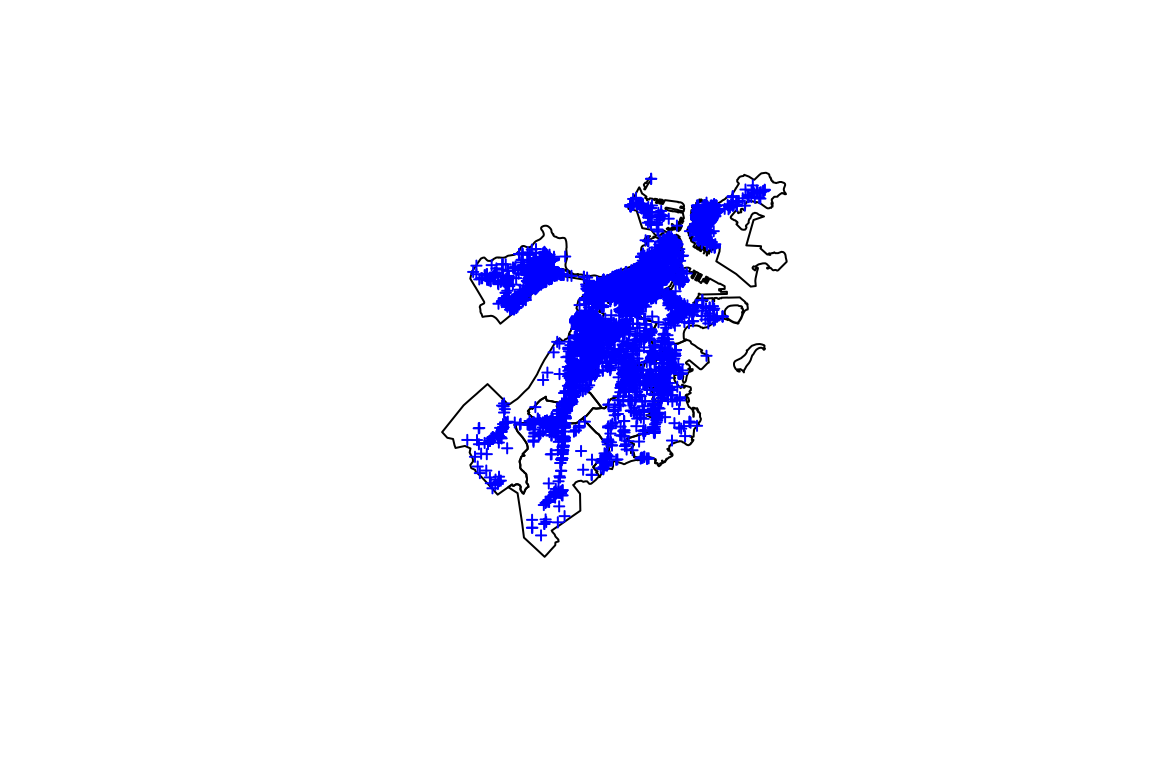This tutorial benefited extensively from several other sources, including but not limited to spatial.org and Nick Eubank’s (here) and Justin de Benedictis Kessner’s (here) great online tutorials.

# How R Sees Spatial Data

In this first section, we will understand how R thinks about spatial data and how to import and export spatial datasets.

First we will load the libraries we will need during this workshop. If you have not installed the packages yet, refer to the pre-workshop instructions.

``````library(sp)
library(rgdal)
library(raster)
library(rgeos)
library(plyr)
library(maptools)
library(RColorBrewer)
library(ggmap)
library(gridExtra)
library(classInt)
library(geosphere)
library(spdep)
library(gmapsdistance)``````

## Make Spatial Data Points

We start with the most basic geometric object, points. Like regular data points in plots, each spatial point needs a pair of x-y coordinates. The only difference is that this time, we pass them to the `SpatialPoints()` function and thus turn these points into a `SpatialPoints` object.

``````plus.coordinates <- rbind(c(1.5, 1.25), c(1.75, 1.25),
c(2.25, 1.25), c(2.5, 1.25), #horizontal
c(2, 1.75), c(2, 1.5),
c(2, 1), c(2, 0.75), #vertical
c(2, 1.25)) #center
first.spatial.points <- SpatialPoints(plus.coordinates) #points converted into spatial object
plot(first.spatial.points)                         ``````To grasp how R sees these points, we can ask use the `summary()` command. The output will show us the class of the object, minimum and maximum coordinates (which will be longitude and latitude values instead of random points in geospatial data), whether the object is projected or not (See below for more info), if projected, which coordinate reference system it uses (CRS), and finally, the total number of points in the data. If you want to reach all the coordinate values, you can use `coordinates()` command.

``summary(first.spatial.points)``
``````## Object of class SpatialPoints
## Coordinates:
##            min  max
## coords.x1 1.50 2.50
## coords.x2 0.75 1.75
## Is projected: NA
## proj4string : [NA]
## Number of points: 9``````
``summary(first.spatial.points)\$class``
``````##  "SpatialPoints"
## attr(,"package")
##  "sp"``````
``summary(first.spatial.points)\$bbox``
``````##            min  max
## coords.x1 1.50 2.50
## coords.x2 0.75 1.75``````
``summary(first.spatial.points)\$is.projected``
``##  NA``
``summary(first.spatial.points)\$proj4string``
``##  NA``
``summary(first.spatial.points)\$npoints``
``##  9``
``coordinates(first.spatial.points)``
``````##       coords.x1 coords.x2
##  [1,]      1.50      1.25
##  [2,]      1.75      1.25
##  [3,]      2.25      1.25
##  [4,]      2.50      1.25
##  [5,]      2.00      1.75
##  [6,]      2.00      1.50
##  [7,]      2.00      1.00
##  [8,]      2.00      0.75
##  [9,]      2.00      1.25``````

The plus we created above is a simple spatial object for now. This `SpatialPoints` object will understand how the coordinates of its points relate to places in the real world and become geospatial through a Coordinate Reference System (CRS), which combines info on the geographic coordinate system and the projection. The CRS of an object can be found through `proj4string()` or the summary of the object as we observed above. The `NA` value means that our SpatialPoints does not have a CRS defined yet. You can also use the `is.projected()` command, which will tell you whether a CRS is defined or not.

``is.projected(first.spatial.points) #see if a projection is defined``
``##  NA``
``````#FALSE if it has a geographic coordinate system but lacks a projection,
#NA if it lacks both. ``````

So we need to define a CRS so that our spatial object becomes a geospatial object. The CRS can be defined by simply passing the reference code for the projection. We’ll use the most common CRS, EPSG:4326, although there are many other possible reference systems: `CRS("+init=EPSG:4326")`. Normally the parameters we needed to define is much longer but fortunately for R to understand it `+init=EPSG:4326` is sufficient, it fills in all the missing parameters.

``CRS("+init=EPSG:4326") #is called with only an EPSG code, R will try to complete all the CRS parameters``
``````## CRS arguments:
##  +init=EPSG:4326 +proj=longlat +datum=WGS84 +no_defs +ellps=WGS84
## +towgs84=0,0,0``````
``````crs.geo <- CRS("+init=EPSG:4326")
proj4string(first.spatial.points) <- crs.geo  #assign a projection to our data
is.projected(first.spatial.points) #check it is defined now``````
``##  FALSE``

This `SpatialPoints` object doesn’t contain any variables at the moment. We can move from a `SpatialPoints` to a `SpatialPointsDataFrame` by adding a `data.frame` of variables to the points. To practice, we’ll add some randomly generated data. Note the `SpatialPoints` will merge with the `data.frame` based on the order of observations, and therefore the number of our spatial points should be equal to the number of rows in the new data.

``````vars <- data.frame(vars1 = rnorm(9), vars2 = c(101:109))
vars``````
``````first.spdf <- SpatialPointsDataFrame(first.spatial.points, vars)
summary(first.spdf)``````
``````## Object of class SpatialPointsDataFrame
## Coordinates:
##            min  max
## coords.x1 1.50 2.50
## coords.x2 0.75 1.75
## Is projected: FALSE
## proj4string :
## [+init=EPSG:4326 +proj=longlat +datum=WGS84 +no_defs +ellps=WGS84
## +towgs84=0,0,0]
## Number of points: 9
## Data attributes:
##      vars1             vars2
##  Min.   :-2.1758   Min.   :101
##  1st Qu.:-1.1690   1st Qu.:103
##  Median :-0.1975   Median :105
##  Mean   :-0.3422   Mean   :105
##  3rd Qu.: 0.4849   3rd Qu.:107
##  Max.   : 0.7958   Max.   :109``````

Say you don’t have a `SpatialPoints` object but only a `data.frame` with two columns indicating longitude and latitude values. `SpatialPointsDataFrame` object can also be created directly from this data frame in one step. You’ll need to specify which columns contain the coordinates by using the `coordinates()` command. We’ll use this method soon to create the `boston.graf` `SpatialPointsDataFrame`.

## SpatialPolygons

Like a `SpatialPoints` consists of points, a `SpatialPolygons` object consists of polygons. To obtain a `SpatialPolygons` object, we need three steps. We’ll first create `Polygon` objects, two-dimensional geometrical shapes, from points. Then we will combine those into `Polygons` objects (Here, we need to give each polygon a unique ID). Finally we will combine `Polygons` into `SpatialPolygons`.

``````#create polygon objects from points.
triangle1 <- Polygon(rbind(c(1, 1), c(2, 1), c(1.5, 0)))
triangle2 <- Polygon(rbind(c(1, 1), c(1.5, 2), c(2, 1)))

#create polygons objects from polygon
t1 <- Polygons(list(triangle1), "triangle1")
t2 <- Polygons(list(triangle2), "triangle2")

#create spatial polygons object from polygons, equal to shapefiles.
first.spatial.polygons <- SpatialPolygons(list(t1, t2))
plot(first.spatial.polygons)``````As with `SpatialPoints`, we can add variables to `SpatialPolygons`. Note that the rownames of the `data.frame` that includes our variables should match the unique IDs of the polygon objects. Ideally the number of our spatial polygons should be equal to the number of rows in the new data so that we don’t have any missing values in our `SpatialPolygonsDataFrame`.

``````vars <- data.frame(attr1 = 1:2, attr2 = 6:5,
row.names = c("triangle2", "triangle1"))
first.spoldf <- SpatialPolygonsDataFrame(first.spatial.polygons, vars)
as.data.frame(first.spoldf) #the rows were re-ordered to match the ID``````
``spplot(first.spoldf)``Remember that in order the `SpatialPoints` object to understand how the coordinates of its points relate to places in the real world, we assigned to it a Coordinate Reference System (CRS). We’ll do the same for this `SpatialPolygons`, using exactly the same method.

``````crs.geo <- CRS("+init=EPSG:4326") #geographical, datum WGS84
proj4string(first.spoldf) <- crs.geo``````

# Importing and Exporting Spatial Data

Of course creating `SpatialPoints` or `SpatialPolygons` by hand is not common. Instead we use existing spatial data by reading them from external sources, the most common among whom is shapefiles.

In order to read shapefiles, which will usually be in form of an ESRI shapefile, we will use `readOGR()` and `writeOGR()` commands. `readOGR()` needs at least the following two arguments: `dsn =`, which asks the path to the folder that contains the files, and `layer =`, which asks the name of the shapefile (without the extension `.shp`).

``````boston <- readOGR(dsn = "BostonData",
layer = "city_council_districts") ``````
``````## OGR data source with driver: ESRI Shapefile
## Source: "BostonData", layer: "city_council_districts"
## with 9 features
## It has 5 fields
## Integer64 fields read as strings:  OBJECTID``````

To understand the shapefile, it’s good to check what it includes using the `str` command. It will show that `boston` is actually a combination of `data`, `coords`, `bbox`, and a `proj4string`. We can call each single attribute either by the corresponding command or by using the `@` command.

``````#str(sf)
bbox(boston) #or boston```bbox``````
``````##         min       max
## x  739594.1  795021.6
## y 2908187.3 2970072.8``````
``coordinates(boston)[1:5, ] #or boston```coords``
``````##       [,1]    [,2]
## 0 787005.1 2961184
## 1 777534.0 2948923
## 2 776281.9 2933061
## 3 769011.6 2930656
## 4 757770.8 2922005``````
``as.data.frame(boston)[1:5, ] #or boston```data``
``proj4string(boston) #orboston```proj4string``
``##  "+proj=lcc +lat_1=41.71666666666667 +lat_2=42.68333333333333 +lat_0=41 +lon_0=-71.5 +x_0=199999.9999999999 +y_0=750000 +datum=NAD83 +units=us-ft +no_defs +ellps=GRS80 +towgs84=0,0,0"``

# Combining Spatial Data with Other Data Sources

## Merging

### Spatial + Non-Spatial

Now we will learn how to merge a Spatial object (`SpatialPolygonsDataFrame`, or `SpatialPointsDataFrame`) with other tabular data, using a common unique identifier. First, load the elections tabular data.

``boston.elections <- read.csv("BostonData/dist_results.csv")``

Now we have i) a `SpatialPolygons` object named `boston`, and ii) a data frame called `boston.elections`, where DISTRICT is the column that contains the unique identifier in `boston`, and district is the column that contains the unique identifier in `boston.elections`.

``````boston <- merge(boston, boston.elections,
by.x = "DISTRICT", by.y = "District")
boston@data[1:5, ] ##check if merged``````

Never merge the `@data` attribute of your Spatial object directly with other data since it may corrupt your data:

``````boston@data <- merge(boston@data, boston.elections,
by.x = "DISTRICT", by.y = "District")``````

### Spatial + Spatial

Before merging two Spatial datasets, the first thing you need to do is to check whether they have the same CRS. Because both of your files should aggree on which exact point on Earth they refer to with a given value. If you try to merge two spatial objects with a different CRS, your results won’t make much sense.

If they don’t have the same CRS, you’ll need to reproject one of your objects. (Reprojecting changes not just the `proj4string` associated with an object, but also all the actual x and y coordinates.)

You can reproject any vector data very simply using the `sptransform()` command, which will need two inputs: i) the object you want to reproject, and ii) the CRS code you want for your reprojection. I’ll show it here with some fake data for now, since we’ll try it with real data later when we create maps.

``````common.crs <- CRS("+init=EPSG:4326")
MyCity.reprojected <- spTransform(MyCity, common.crs)``````

Since our ultimate goal is to make sure both of your spatial objects have the same CRS, you can also retrieve the CRS from one spatial object with the `proj4string()` command, store it, and reproject your second spatial object with this CRS you stored. So if you have two objects named MyCityA and MyCityB, the code will look as follows:

``````common.crs <- CRS(proj4string(MyCityB))
MyCityA.reprojected <- spTransform(MyCityA, common.crs)``````

## Spatial Merges

So far we have used a common unique identifier to merge, as with the regular datasets. We can also use the geolocation to merge. Now we’re going to work with Boston municipal service requests for graffiti removal.

We already know the geolocation of graffitis but we don’t know in which district of Boston they are located. So we’ll just overlay the graffitis with our Boston map, a `SpatialPolygons` object, using the `over()` command, which will match each graffiti with a district. After the match we’ll combine the two data using the `spCbind()` command.

Let’s start by loading our graffiti `data.frame` and turning it into a `SpatialPointsDataFrame` so that R understands that it is a geospatial file where each graffiti shows an exact location (longitude and latitude):

``````boston.requests <- read.csv("BostonData/graffiti.csv")
boston.graf <- SpatialPointsDataFrame(coords = cbind(boston.requests\$LONGITUDE,
boston.requests\$LATITUDE),
data = boston.requests,
proj4string = CRS("+proj=longlat"))``````

Next we need to make sure the Boston map has the same projection as our spatial point data frame:

``boston <- spTransform(boston, CRS("+proj=longlat"))``

Now for every graffiti, I want to get information about their district. I will use the `over()` command. The command basically asks R to return the row number of the observation in Data 2 that intersects with any given observation in Data 1.

``````boston.districts <- over(boston.graf, boston)
boston.districts[1:5, ] ##check if overlaid``````

Note that `over` only returns the relevant row of Data 2 for each point. If `boston` did not have any data, we could just get the index of the intersecting polygon for each graffiti. If we just want to know the polygon index, we can use the `geometry()` command to remove the rest of the `boston.graf` data:

``````boston.districts2 <- over(boston.graf, geometry(boston))
boston.districts2[1:5] ##check if overlaid``````
``````## 1 2 3 4 5
## 3 3 6 9 1``````

Finally we use the `spCbind()` command to merge the two spatial objects.

``````boston2 <- spCbind(boston.graf, boston.districts)
names(boston2)``````
``````##   "CASE_ENQUIRY_ID"                "OPEN_DT"
##   "CLOSED_DT"                      "TIME_DIFF"
##   "CASE_STATUS"                    "CLOSURE_REASON"
##   "CASE_TITLE"                     "SUBJECT"
##   "REASON"                         "TYPE"
##  "QUEUE"                          "Department"
##  "Location"                       "neighborhood"
##  "neighborhood_services_district" "LOCATION_STREET_NAME"
##  "LOCATION_ZIPCODE"               "LATITUDE"
##  "LONGITUDE"                      "Source"
##  "completion.time"                "DISTRICT"
##  "OBJECTID"                       "Councillor"
##  "SHAPE_area"                     "SHAPE_len"
##  "Winning_Perc"                   "Runner_Perc"
##  "MOV"``````

# Making Maps

## Making Maps with `plot()`

Now that we merged our data with other data sources, non-spatial or spatial, we are ready to create maps. For most plots we can simply use the `plot()` command. Let’s first create a very simple plot where we overlay the Boston districts and graffiti locations:

``````plot(boston)Another useful plotting method is coloring the polygons based on the values in one of the attributes. For instance, since we have already merged the Boston districts with the election results, we can color the districts based on the margin of victories in the city council elections. These maps, in which areas are shaded in proportion to the measurement of the statistical variable being shown on the map, are called choropleth maps.

One important step in creating the plot is getting the color codes we’ll use. For this you’ll i) choose n - the number of cuts you want to use, ii) obtain the quantile values that indicate the cutoff points, iii) pick a palette you like from amongst the options available in the `brewer.pal()` function. Now you are ready to obtain your color codes using the `findColours()` function. Note that different palettes have different limits on the maximum and minimum number of cuts allowed.

``````#determine the color codes
var <- as.numeric(boston\$MOV)
nclr <- 5
plotclr <- brewer.pal(nclr,"Blues")
class <- classIntervals(var, nclr, style="quantile")
colcode <- findColours(class, plotclr)``````

Now you can just pass these color codes to `plot()`:

``````#plot:
plot(boston, col = colcode, border=NA) #remove the borders since looks prettier
legend(x = "bottomright",
fill = attr(colcode,"palette"), #set the legend colors
bty = "n", #remove the around the legend
legend = names(attr(colcode, "table")), #show the quantile values
title = "MOV Quantile",
cex = 0.6) #adjust legend size ``````